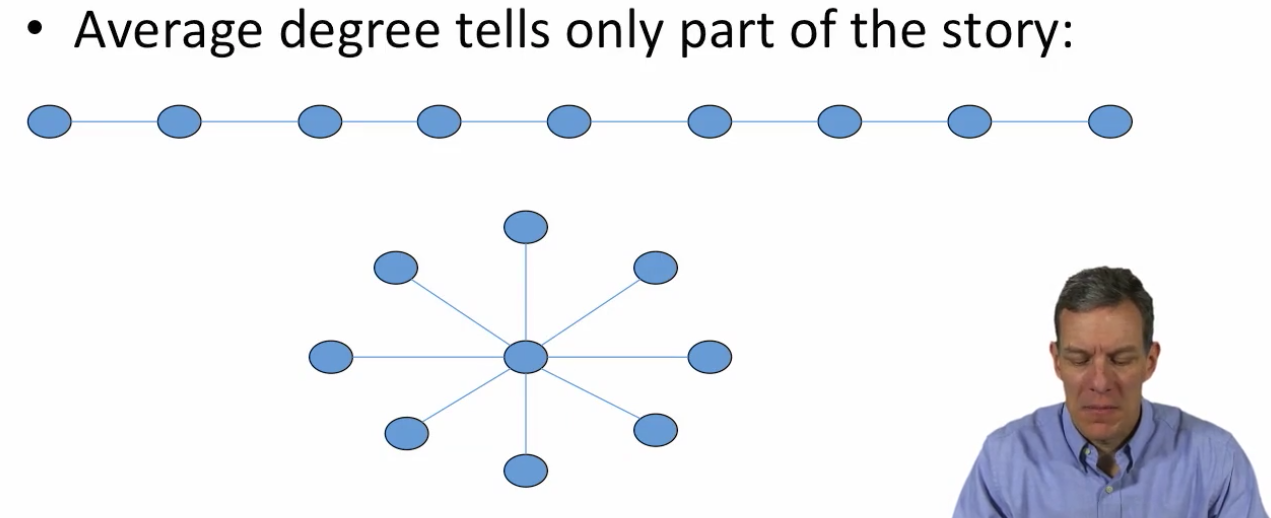# Degree Distribution, G(n, p)¶

• probability that node has d links is binomial $$\frac{(n-1)!}{d!(n-d-1)!} p^d (1-p)^{n-d-1}$$

• Large n, small p, this is approximately a Poisson distribution $$\frac{(n-1)^d}{d!} p^d e^{-(n-1)p}$$

• why Poisson? If you want to approximate this if you want to approximate this formula(binomial formula) for large n and relatively small p.

• $(1-p)^{n-d-1} \rightarrow e^{-(n-1)p}$
• $\frac{(n-1)!}{d!(n-d-1)!} \rightarrow \frac{(n-1)^d}{d!}$
• $\frac{(n-1)!}{(n-d-1)!} \rightarrow (n-1)^d$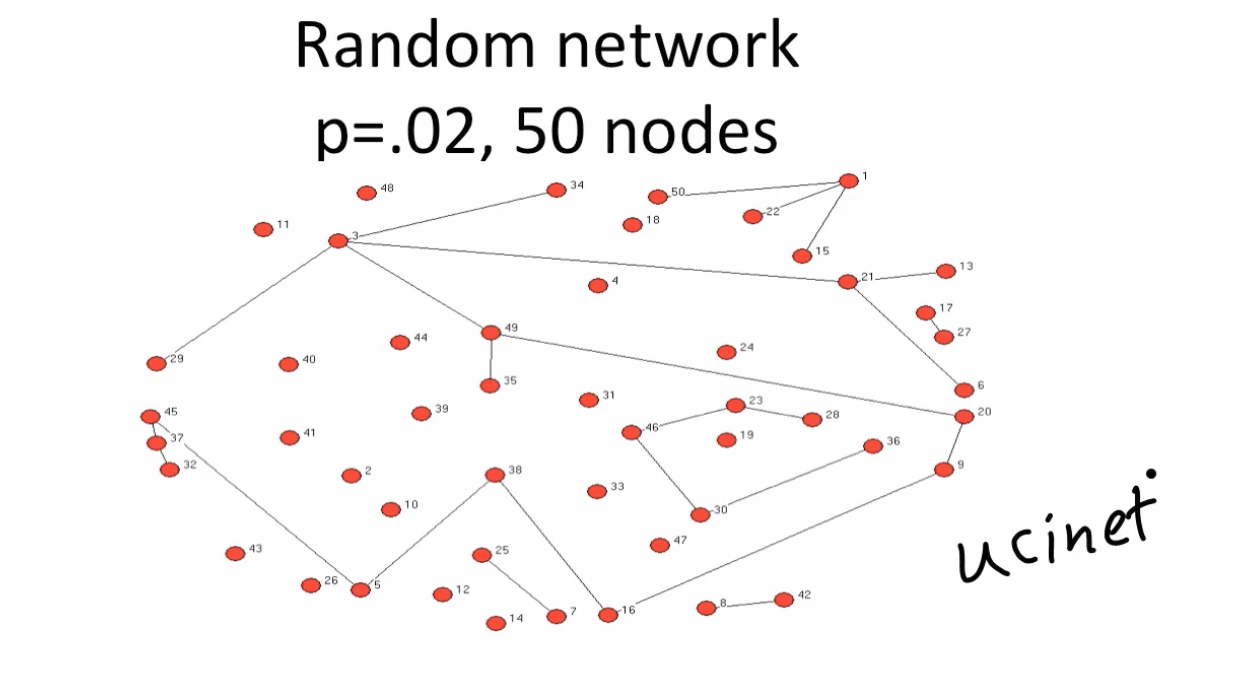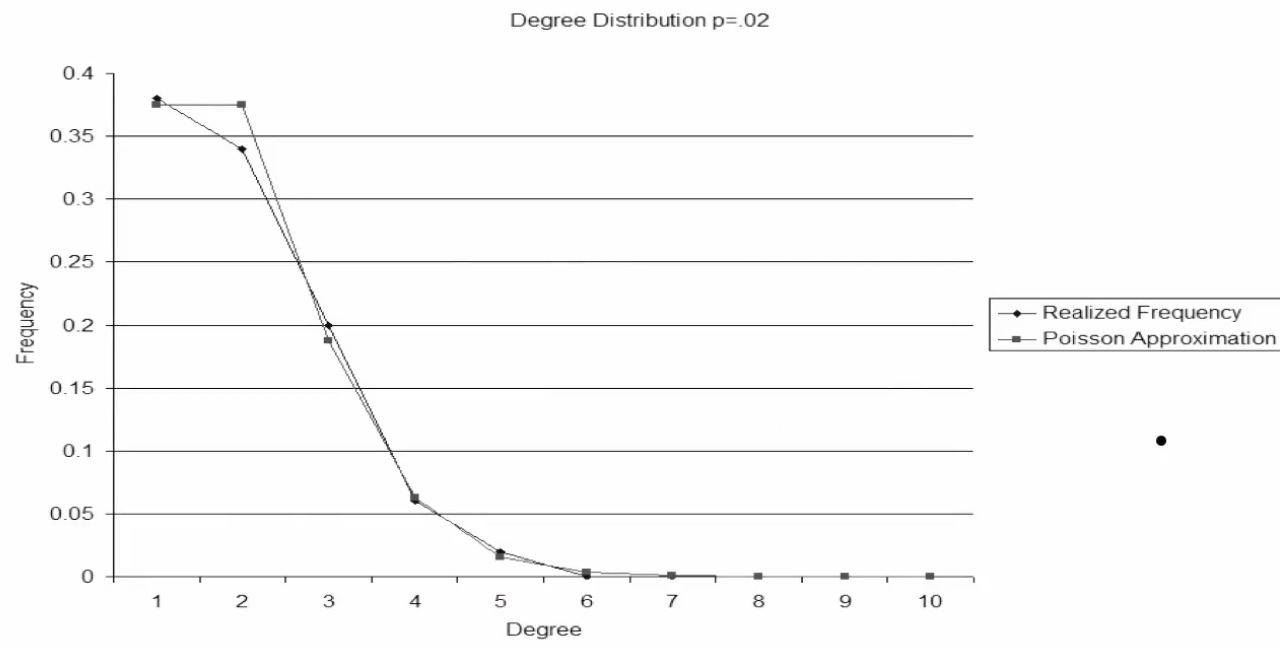Note:

• many isolated nodes
• several components
• no component has more than a small fraction of the nodes, just starting to see one large one emerge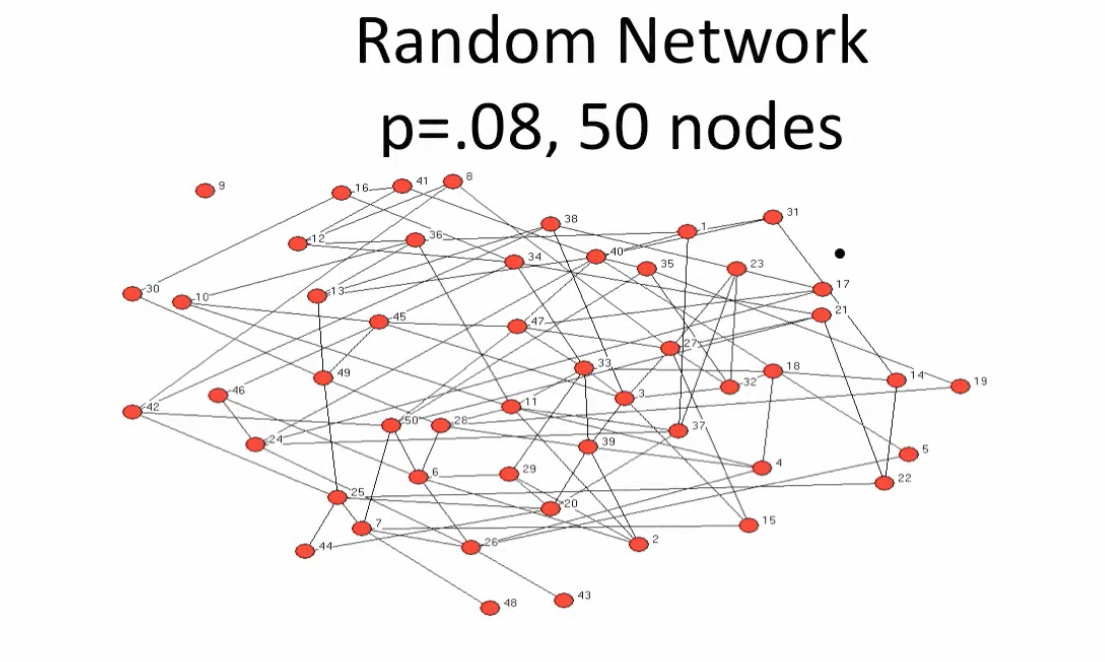# Distribution of links per node: Fat tails (Price 1969)¶

• More high and low degree nodes than predicted at random
• Citation Networks - too many with 0 citations, too many with high numbers of of citations to have citations drawn at random
• "Fat tails" compared to random network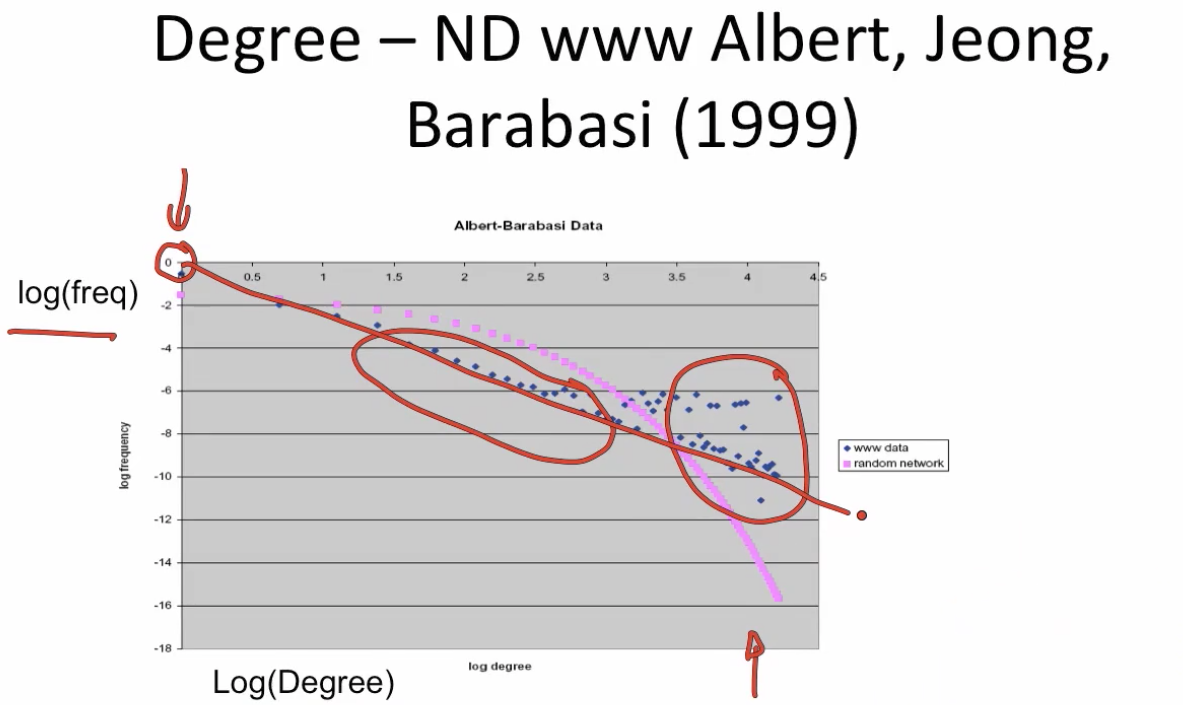# Scale Free Distributions¶

• $P(d) = cd^{-a}$

• $log(P(d)) = log(c) - alog(d)$